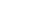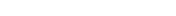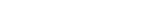xEncyclopedia
In mathematical numeral systems
Numeral system
A numeral system is a writing system for expressing numbers, that is a mathematical notation for representing numbers of a given set, using graphemes or symbols in a consistent manner....

, the base or radix for the simplest case is the number of unique digits
Numerical digit
A digit is a symbol used in combinations to represent numbers in positional numeral systems. The name "digit" comes from the fact that the 10 digits of the hands correspond to the 10 symbols of the common base 10 number system, i.e...

, including zero, that a positional
Positional notation
Positional notation or place-value notation is a method of representing or encoding numbers. Positional notation is distinguished from other notations for its use of the same symbol for the different orders of magnitude...

numeral system
Numeral system
A numeral system is a writing system for expressing numbers, that is a mathematical notation for representing numbers of a given set, using graphemes or symbols in a consistent manner....

uses to represent numbers. For example, for the decimal
Decimal
The decimal numeral system has ten as its base. It is the numerical base most widely used by modern civilizations....

system (the most common system in use today) the radix is ten, because it uses the ten digits from 0 through 9.

In any numeral system, the base is written as "10". In a base ten numeral system, "10" represents the number ten; in a base two system, "10" represents the number two.

## Etymology

Radix is a Latin word for "root". Root can be considered a synonym for base in the arithmetical sense.

## In numeral systems

In the system with radix 13, for example, a string of digits such as 398 denotes the decimal number. More generally, in a system with radix b (b > 1), a string of digitsdenotes the decimal number.

Commonly used numeral systems include:
• The decimal system
Decimal
The decimal numeral system has ten as its base. It is the numerical base most widely used by modern civilizations....

, the most used system of numbers in the world, is used in arithmetic. Its ten digits are "0–9".
• The duodecimal (dozenal) system
Duodecimal
The duodecimal system is a positional notation numeral system using twelve as its base. In this system, the number ten may be written as 'A', 'T' or 'X', and the number eleven as 'B' or 'E'...

, which is base 12, is often used due to divisibility by 2, 3, 4 and 6. It was traditionally used as part of quantities expressed in dozens
Dozen
A dozen is a grouping of approximately twelve. The dozen may be one of the earliest primitive groupings, perhaps because there are approximately a dozen cycles of the moon or months in a cycle of the sun or year...

and grosses
Gross (unit)
A gross is equal to a dozen dozen, i.e. 12 × 12 = 144.It can be used in duodecimal counting. The use of gross likely originated from the fact that 144 can be counted on the fingers using the fingertips and first two joints of each finger when marked by the thumb of one hand. The other hand...

.
• The binary numeral system
Binary numeral system
The binary numeral system, or base-2 number system, represents numeric values using two symbols, 0 and 1. More specifically, the usual base-2 system is a positional notation with a radix of 2...

, used internally by nearly all computer
Computer
A computer is a programmable machine designed to sequentially and automatically carry out a sequence of arithmetic or logical operations. The particular sequence of operations can be changed readily, allowing the computer to solve more than one kind of problem...

s, is base two. The two digits are "0" and "1", expressed by different electric charge
Electric charge
Electric charge is a physical property of matter that causes it to experience a force when near other electrically charged matter. Electric charge comes in two types, called positive and negative. Two positively charged substances, or objects, experience a mutual repulsive force, as do two...

s.
In mathematics and computer science, hexadecimal is a positional numeral system with a radix, or base, of 16. It uses sixteen distinct symbols, most often the symbols 0–9 to represent values zero to nine, and A, B, C, D, E, F to represent values ten to fifteen...

, which is base 16, is often used in computing. The sixteen digits are "0–9" followed by "A–F".
• The octal system
Octal
The octal numeral system, or oct for short, is the base-8 number system, and uses the digits 0 to 7. Numerals can be made from binary numerals by grouping consecutive binary digits into groups of three...

, which is base 8, is occasionally used in computing. The eight digits are "0–7".
• Base 64
Base64
Base64 is a group of similar encoding schemes that represent binary data in an ASCII string format by translating it into a radix-64 representation...

is also occasionally used in computing, using as digits "A–Z", "a–z", "0–9", plus two more characters, often "+" and "/".

The octal, hexadecimal and base-64 systems are often used in computing because of their ease as shorthand for binary. For example, every hexadecimal digit has an equivalent 4 digit binary number.

Radices are usually natural numbers. However, more sophisticated positional systems are possible, e.g. golden ratio base
Golden ratio base
Golden ratio base is a non-integer positional numeral system that uses the golden ratio as its base. It is sometimes referred to as base-φ, golden mean base, phi-base, or, colloquially, phinary...

(whose radix is a non-integer algebraic number
Algebraic number
In mathematics, an algebraic number is a number that is a root of a non-zero polynomial in one variable with rational coefficients. Numbers such as π that are not algebraic are said to be transcendental; almost all real numbers are transcendental...

), and negative base
Negative base
A negative base may be used to construct a non-standard positional numeral system. Like other place-value systems, each position holds multiples of the appropriate power of the system's base; but that base is negative—that is to say, the base \scriptstyle b is equal to \scriptstyle -r for some...

## In exponentiation

In exponentiation
Exponentiation
Exponentiation is a mathematical operation, written as an, involving two numbers, the base a and the exponent n...

, the base refers to the number b in an expression of the form bn. The number n is called the exponent and the expression is known formally as exponentiation of b by n or the exponential of n with base b. It is more commonly expressed as "the nth power of b", "b to the nth power" or "b to the power n".

When the nth power of b equals a number a, or  a = bn , then b is called an "nth root
Nth root
In mathematics, the nth root of a number x is a number r which, when raised to the power of n, equals xr^n = x,where n is the degree of the root...

" of a. The term power strictly refers to the entire expression, but is sometimes used to refer to the exponent.

The inverse function
Inverse function
In mathematics, an inverse function is a function that undoes another function: If an input x into the function ƒ produces an output y, then putting y into the inverse function g produces the output x, and vice versa. i.e., ƒ=y, and g=x...

to exponentiation with base b (when it is well-defined
Well-defined
In mathematics, well-definition is a mathematical or logical definition of a certain concept or object which uses a set of base axioms in an entirely unambiguous way and satisfies the properties it is required to satisfy. Usually definitions are stated unambiguously, and it is clear they satisfy...

) is called the logarithm
Logarithm
The logarithm of a number is the exponent by which another fixed value, the base, has to be raised to produce that number. For example, the logarithm of 1000 to base 10 is 3, because 1000 is 10 to the power 3: More generally, if x = by, then y is the logarithm of x to base b, and is written...

to base b, denoted logb. Thus: